### KA_IX

• 2976 主题
• 3026 帖子
• 8542 积分
• 身份：LV6 初级工程师
•• E币：2639

# 贴片电阻值的读数方法（E96标注法，E24标注法）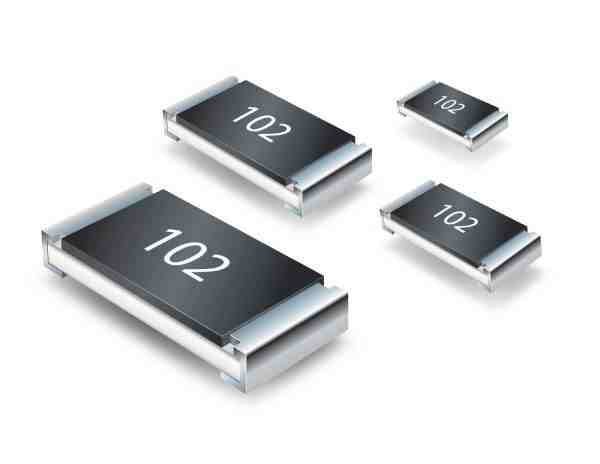E24标注法对于E24标注法如果是三位数字，则前两位是电阻值的有效数字，第三位是10的倍率，比如数字是103的电阻阻值就是10*10^3 = 10K，而470则是47*10^0 = 47欧，而不是470欧。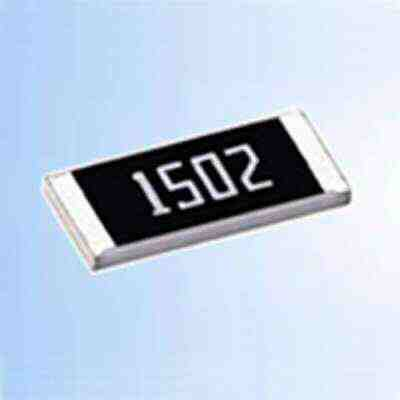E96标注法对于E96标注法一般常规的是使用4位数字表示阻值，前三位数字表示电阻的有效值，最后一位则是10的倍率，比如1003，前三位100是有效值，就是一位是3是10^3，所1003=100*10^3=100K，如果是4700则前三位是470，就后一位的0表示10的0次方，所以4700=470欧，而不是4.7K。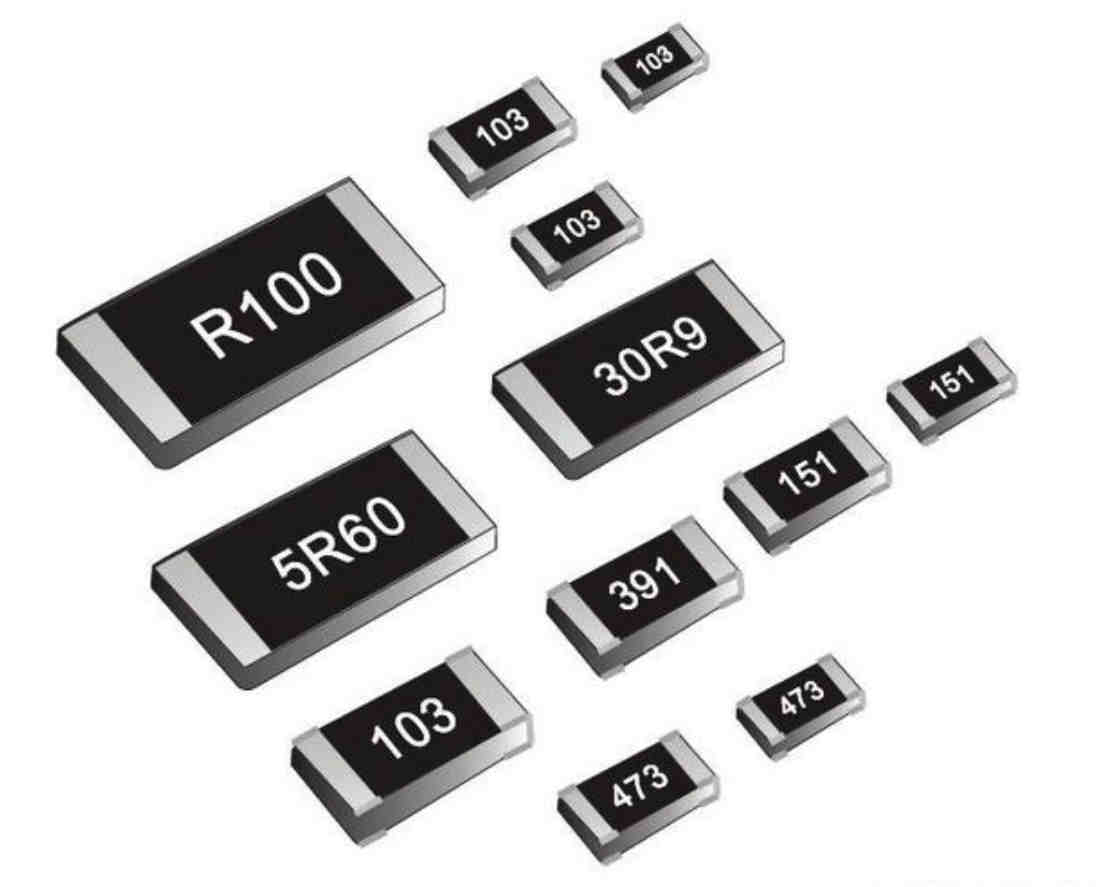1R00 = 1.00Ω
5R10 = 5.10Ω
R200 = 0.200Ω
R007 = 7.00mΩ
4m70 = 4.70mΩ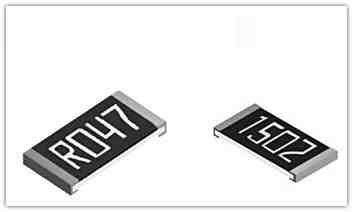E96标注法还有一种特殊情况，就是称为MulTIplier Code标注法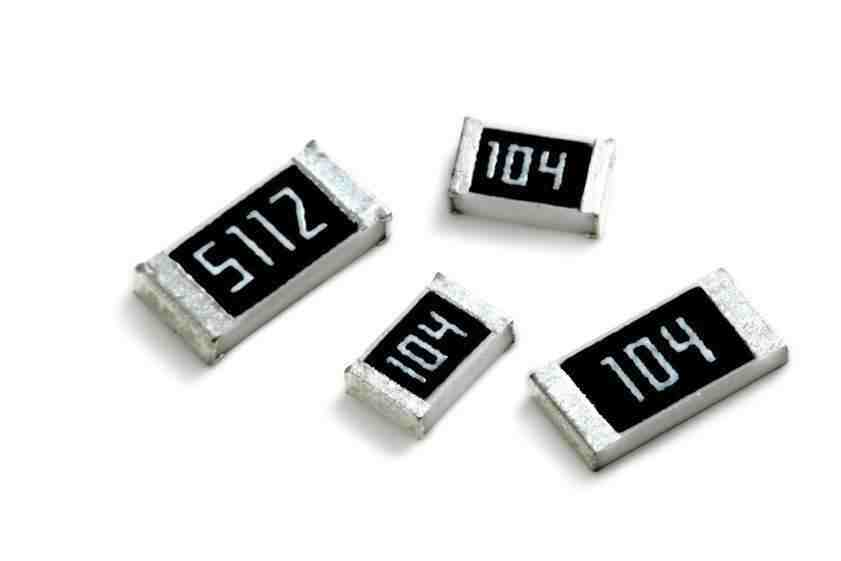01C = 100*10^2=10KΩ
50D = 324*10^3=324kΩ

0
0